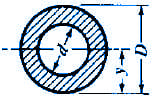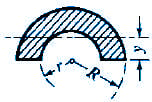Moment of Inertia, Section Modulus, Radii of Gyration Equations
Circular, Eccentric Shapes

Moment of Inertia, Section Modulus, Radii of Gyration Equations Circular, Eccentric Shapes

 A = Area (in2, mm2) I = Moment of Inertia (in4, mm4) Gr = Radius of Gyration = (in, mm) y = Distance of Axis to Extreme Fiber (in, mm)

 SectionOpen Section Properties Case 17 CalculatorOpen Section Properties Case 18 CalculatorOpen Section Properties Case 19 Calculator Moment of InertiaRadius of GyrationAreaySectionOpen Section Properties Case 20 CalculatorOpen Section Properties Case 21 CalculatorOpen Section Properties Case 22 Calculator Moment of InertiaRadius of GyrationAreayRelated:Membership Register | LoginHomeEngineering Book StoreEngineering ForumExcel App. DownloadsOnline Books & ManualsEngineering NewsEngineering VideosEngineering CalculatorsEngineering ToolboxGD&T Training Geometric Dimensioning TolerancingDFM DFA TrainingTraining Online EngineeringAdvertising CenterCopyright Notice